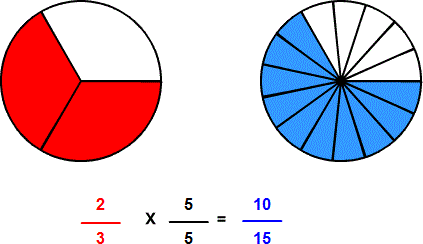# Rename to higher terms with circle models

## Rename fractions to higher terms is pictured with circle models.

RENAME to HIGHER TERMS
CORRECT:
ATTEMPTS:
SCORE:
PERCENT
NUMERATOR
LOWER TERMS
HIGHER TERMS

# RENAME TO HIGHER TERMS WITH CIRCLE MODELS INSTRUCTIONS

Follow the directions in the dialog box after pressing the <START> button. The <EXPLAIN> button may then be pressed to see how to do the example.

Rename to Higher Terms uses circles to demonstrate how a fraction can be renamed to higher terms .

The following equivalent fractions were made by Rename to Higher Terms with Circles Designer:When the program starts, you will be shown a fractional part of a circle. The denominator of an equivalent fraction will be given. You are to find the numerator of the fraction with the given denominator.

The equivalent fraction can be written by multiplying the numerator and denominator of the first fraction by the same number. In the example above, both the numerator and denominator are multiplied by 5 Actually, we are multiplying the fraction by 5/5, a form of one. One (1) is called the multiplicative identity because multiplying a number by one or some form of one does not change the value of the number.

The 5 is arrived at by asking, "What times the denominator 3 equals 15?". Since the denominator is multiplied by 5 then the numerator must be multiplied by 5.

When a correct numerator is entered, a second circle will appear. The two circles represent the same amount. Although there are more parts in the second circle, the parts are smaller, making the two fractions equivalent. The second number is in higher terms.

For more instruction on renaming to higher terms go to How To Rename to Higher Terms.

After you enter first example you may press the <REPORT> button. This report will give same results as on the dialog box. The report will ask for your name but you may submit a code for your name. The report may be printed or e-mailed.